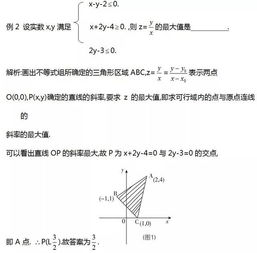高考线性规划知识点总结(高中数学线性规划知识)

1.高中数学 线性规划 知识

1.二元一次不等式表示平面区域.

(1)一般地，二元一次不等式在平面直角坐标系中表示直线某一

（半平面）包括边界线.

(2)判定不等式（或）所表示的平面区域时，只要在直线

(3)由几个不等式组成的不等式组表示的平面区域是各个不等式所表示的平面区域的公共部分.

2.线性规划问题的图解法：

(1)基本概念

(2)用图解法解决线性规划问题的一般步骤

①设出所求的未知数；②列出约束条件（即不等式组）；③建立目标函数；

④作出可行域； ⑤运用图解法求出最优解.

3.解法归类：

(1)图解法；（2）列表法；（3）待定系数法；（4）调整优值法；（5）打网格线法；

(6)交点定界法。

4.高中数学线性规划

x+y=5 (1) 2x-y=4 (2) y=x+1 (3) y=0 (4) x+y≤5 (1') 2x-y≤4 (2') y≤x+1 (3') y≥0 (4') case 1: (1) and (2) x+y=5 (1) 2x-y=4 (2) (1)+(2) x=3 from (1) =>y=2 (x,y)=(3,2) 满足 y≤x+1 (3') and y≥0 (4') z=2x+y z(3,2) = 2(3) +2 =8 case 2: (1) and (3) x+y=5 (1) y=x+1 (3) from (1) and (3) x+x+1 =5 x=2 from (1) =>y=3 (x,y)=(2,3) 满足 2x-y≤4 (2') and y≥0 (4') z=2x+y z(2,3) = 2(2) +3 =7 case 3: (1) and (4) x+y=5 (1) y=0 (4) from (1) and (4) x=5 (x,y)=(5,0) 不满足 2x-y≤4 (2') case 3： 舍去 case 4: (2) and (3) 2x-y=4 (2) y=x+1 (3) from (2),(3) 2x-(x+1) =4 x=5 from (3) =>y=6 (x,y)=(5,6) 不满足 x+y≤5 (1') case 4： 舍去 case 5: (2) and (4) 2x-y=4 (2) y=0 (4) from (2) and (4) x=2 (x,y)=(2,0) 满足 x+y≤5 (1') and y≤x+1 (3') z=2x+y z(2,0) = 2(2) +0 =4 case 6: (3) and (4) y=x+1 (3) y=0 (4) from (3) and (4) x=1 (x,y)=(1,0) 满足 x+y≤5 (1') and 2x-y≤4 (2') z=2x+y z(1,0) = 2(1) +0 =2 ie max z =case 1 =z(3,2) =8。